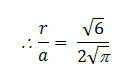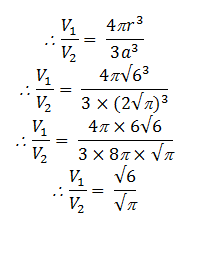Newbie

# A sphere and a cube have the same surface area. Show that the ratio of the volume of the sphere to that of the cube is root 6 : root pi

• 0

This is the Important question of class 10 Based on Mensuration Chapter of M.L Aggarwal book for ICSE BOARD.
Here A sphere and a cube have the same surface area.
Show that the ratio of the volume of the sphere to that of the cube is √6 :√π
This is the Question Number 09, Exercise 17.3 of M.L Aggarwal.

Share

1.Let r be the radius of the sphere and a be the side of the cube.

Surface area of sphere = 4r2

Surface area of cube = 6a2

Given sphere and cube has same surface area.

4r2 = 6a2

r2/a2 = 6/4Volume of the sphere, V= (4/3)r3

Volume of the cube, V= a3Hence proved.

• 1